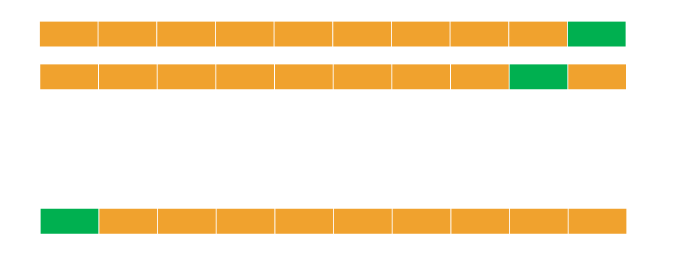• No products in the cart.

# 204.4.11 K-fold Cross Validation

##### Understanding and Practicing K-fold Cross validation
`Link to the previous post : https://statinfer.com/204-4-10-cross-validation/`

### Ten-fold Cross – Validation

• Divide the data into 10 parts(randomly)
• Use 9 parts as training data(90%) and the tenth part as holdout data(10%)
• We can repeat this process 10 times
• Build 10 models, find average error on 10 holdout samples. This gives us an idea on testing error.### K-fold Cross Validation

• A generalization of cross validation.
• Divide the whole dataset into k equal parts
• Use kth part of the data as the holdout sample, use remaining k-1 parts of the data as training data.
• Repeat this K times, build K models. The average error on holdout sample gives us an idea on the testing error.
• Which model to choose?
• Choose the model with least error and least complexity.
• Or the model with less than average error and simple (less parameters).
• Finally use complete data and build a model with the chosen number of parameters.
• Note: Its better to choose K between 5 to 10. Which gives 80% to 90% training data and rest 20% to 10% is holdout data.

### Practice : K-fold Cross Validation

• Build a tree model on the fiber bits data.
• Try to build the best model by making all the possible adjustments to the parameters.
• What is the accuracy of the above model?
• Perform 10 -fold cross validation. What is the final accuracy?
• Perform 20 -fold cross validation. What is the final accuracy?
• What can be the expected accuracy on the unknown dataset?

Solution

In :
```##Defining the model parameters
tree_KF = tree.DecisionTreeClassifier(criterion='gini',
splitter='best',
max_depth=30,
min_samples_split=30,
min_samples_leaf=30,
max_leaf_nodes=60)
```
In :
```#Importing kfold from cross_validation
from sklearn.cross_validation import KFold
```
In :
```#Simple K-Fold cross validation. 10 folds.
kfold = KFold(len(Fiber_df), n_folds=10)
```
In :
```## Checking the accuracy of model on 10-folds
from sklearn import cross_validation
score10 = cross_validation.cross_val_score(tree_KF,X, y,cv=kfold)
score10
```
Out:
```array([ 0.8358,  0.703 ,  0.6184,  0.8047,  0.8385,  0.7994,  0.7675,
0.7507,  0.7913,  0.7206])```
In :
```#Mean accuracy of 10-fold
score10.mean()
```
Out:
`0.76299000000000006`
In :
```#Simple K-Fold cross validation. 20 folds.
kfold = KFold(len(Fiber_df), n_folds=20)
```
In :
```#Accuracy score of 20-fold model
score20 = cross_validation.cross_val_score(tree_KF,X, y,cv=kfold)
score20
```
Out:
```array([ 0.9048,  0.781 ,  0.8288,  0.612 ,  0.283 ,  0.6676,  0.9226,
0.7482,  0.907 ,  0.7866,  0.6784,  0.866 ,  0.8788,  0.911 ,
0.925 ,  0.7318,  0.9724,  0.7502,  0.6954,  0.7456])```
In :
```#Mean accuracy of 20-fold
score20.mean()
```
Out:
`0.77981`

With 10-fold kross validation we can expect Accuracy : 76.29%.

With 20-fold kross validation we can expect Accuracy : 77.98%.

The next post is about bootstrap cross validation.

Link to the next post : https://statinfer.com/204-4-12-bootstrap-cross-validation/

21st June 2017
0 responses on "204.4.11 K-fold Cross Validation"